Courses
Courses for Kids
Free study material
Offline Centres
More

# Mechanical Properties of Solids Class 11 Notes CBSE Physics Chapter 9 (Free PDF Download)Last updated date: 06th Dec 2023
Total views: 630k
Views today: 18.29k

## Revision Notes for CBSE Class 11 Physics Chapter 9 (Mechanical Properties of Solids) - Free PDF Download

The notes of mechanical properties of solids class 11 are available on Vedantu in PDF format and students can download it for free. Mechanical properties of Solids come under Unit VII- Properties of Bulk Matter. This entire unit is very important from an examination point of view and carries a total weightage of 20 marks.

This chapter talks about various laws and concepts around solid bodies. The chapter is theoretically dense, so to memorize them well students should understand the concepts first. To strengthen the understanding, physics class 11 chapter 9 notes include examples to explain the concepts clearly and logically. Students are advised to go through the notes of mechanical properties of solids class 11 regularly to maximize retention of the concepts and examples.

Also, check CBSE Class 11 Physics revision notes for other chapters:

Mechanical Properties of Solids Chapter-Related Important Study Materials
It is a curated compilation of relevant online resources that complement and expand upon the content covered in a specific chapter. Explore these links to access additional readings, explanatory videos, practice exercises, and other valuable materials that enhance your understanding of the chapter's subject matter.

 Mechanical Properties of Solids Related Other Study Materials Mechanical Properties of Solids Important Questions Mechanical Properties of Solids NCERT Solutions Mechanical Properties of Solids NCERT Exemplar Mechanical Properties of Solids NCERT Formulas

## Mechanical Properties of Solids Class 11 Notes Physics - Basic Subjective Questions

### Section – A (1 Mark Questions)

1. Why does spring balance show wrong readings after they have been used for a long time?

Ans. Because of elastic fatigue.

2. What is an elastomer?

Ans. It is a substance that can be elastically stretched to large values of strain.

3. Give two examples which are nearly perfectly plastic material.

Ans. Putty and paraffin wax.

4. What is the Yield point on a graph drawn between stress-strain?

Ans. Yield point is the point, beyond which the wire starts showing increase in strain without any increase in stress.

5. The stress versus strain graphs for wires of two materials A and B are as shown in the figure. If YA and YB are the Young’s modulii of the materials, then find the relation between Yand YB .Ans. $\dfrac{Y_{A}}{Y_{B}}=\dfrac{tan\;60^{\circ}}{tan\;30^{\circ}}=\dfrac{\sqrt{3}}{\frac{1}{\sqrt{3}}}=3$

$Y_{A}=3Y_{B}$

### Section – B (2 Marks Questions)

6. A body of mass 1 kg is attached to one end of a wire and rotated in a horizontal circle of diameter 40 cm with a constant speed of 2 m/s. What is the area of cross-section of the wire if the stress developed in the wire is $5\times 10^{6}N/m^{2}$ ?

Ans. $F=T=\dfrac{mv^{2}}{r}=\dfrac{1\times 4}{0\cdot 2}=20N$

$Stress=\dfrac{F}{A}$

$\therefore A=\dfrac{F}{Stress}=\dfrac{20}{5\times 10^{6}}=4\times 10^{-6}m^{2}=4mm^{2}$

7. The radii of two wires of the same material are in the ratio 2 : 1. If the wires are stretched by equal forces, find the ratio of the stresses produced in them.

Ans. $Stress=\dfrac{F}{A}$

$\dfrac{S_{1}}{S_{2}}=\dfrac{\dfrac{F}{A_{1}}}{\dfrac{F}{A_{2}}}=\dfrac{A_{2}}{A_{1}}$

$\Rightarrow \dfrac{S_{1}}{S_{2}}=\left ( \dfrac{r_{2}}{r_{1}} \right )^{2}=\left ( \dfrac{1}{2} \right )^{2}$

$\therefore \dfrac{S_{1}}{S_{2}}=\dfrac{1}{4}$

8. The face EFGH of the cube shown in the figure is displaced 2 mm parallel to itself when forces of 5 × 105 N each are applied on the lower and upper faces. The lower face is fixed. Then find the strain produced in the cube.Ans. Shear strain $=\dfrac{X}{l}=\dfrac{2\times 10^{-3}}{4\times 10^{-2}}=0\cdot 05$

9. For a given material the Young’s modulus is 2.4 times that of rigidity modulus, then find the Poisson’s ratio.

Ans. $Y=2\cdot 4\eta$

$Y=2\eta (1+\sigma )$

$2\cdot 4\eta =2\eta (1+\sigma )$

$1\cdot 2=(1+\sigma )$

$\sigma =0\cdot 2$

10. A wire is stretched by 5 mm when it is pulled by a certain force. If the wire of same material but of double the length and double the diameter be stretched by the same force, then find the elongation in wire.

Ans. $dl=\dfrac{Fl}{AY}$

$dl\varpropto\dfrac{l}{r^{2}}$

$\dfrac{dl_{1}}{dl_{2}}=\dfrac{l_{1}r_{2}^{2}}{l_{2}r_{1}^{2}}$

$\dfrac{5}{dl_{2}}=\dfrac{l(2r)^{2}}{2lr^{2}}$

$\dfrac{5}{dl_{2}}=\dfrac{4}{2}=2$

$dl_{2}=\dfrac{5}{2}=2\cdot 5mm$

## PDF Summary - Class 11 Physics Mechanical Properties of Solids Notes (Chapter 9)

### 1. Introduction:

A rigid body refers to a hard solid object having a definite shape and size. However, in reality, bodies can be stretched, compressed and bent. Even the strongest rigid steel bar can be deformed when a sufficiently large external force is applied on it. This suggests that solid bodies are not perfectly rigid. Solids have a definite shape and size. In order to make a change (or deform) their shapes or sizes, a force is always required.

### 2. Deforming Force:

A deforming force can be defined as a force that produces a change in the configuration (size or shape) of the object on application.

### 3. Elasticity:

Elasticity refers to the property of an object by virtue of which it regains its original configuration after having the deforming force removed. For instance, when we stretch a rubber band and release it, it snaps back to its original shape and length.

### 4. Perfectly Elastic Body:

The bodies which have the capability to regain their original configuration immediately and completely after having the deforming force removed are termed perfectly elastic bodies. Quartz fibre can be considered as a perfectly elastic body.

### 5. Plasticity:

When a body does not have the capability to regain its original size and shape completely and immediately after having the deforming force removed, it is called a plastic body and this property is termed as plasticity.

### 6. Perfectly Plastic Body:

A body that does not regain its original configuration at all on the removal of deforming force is known as a perfectly plastic body. Putty and paraffin wax can be considered nearly perfectly plastic bodies.

### 7. Stress:

When an object gets deformed under the action of an external force, then at each section of the object, stress (an internal reaction force) is produced, which tends to restore the body into its original state.

#### 7.1 Definition:

The internal restoring force produced per unit area of the cross-section of the deformed object is termed stress.

#### 7.2  Mathematical Form:

$\text{Stress}=\frac{\text{Applied Force}}{\text{Area}}$

Its unit is $\text{N/}{{\text{m}}^{2}}$ or pascal (Pa).

Its dimensional formula is $\left[ \text{M}{{\text{L}}^{-1}}{{\text{T}}^{-2}} \right]$.

#### 7.3 Types of Stress:

Three different types of stress are known. They are:

#### 1.Longitudinal Stress:

When a deforming force is applied normal to the area of a cross-section, then the stress is termed as longitudinal stress or normal stress. It is further differentiated into two kinds:

1. Tensile Stress: When there is an increase in length of the object under the effect of applied force, then the stress is termed as tensile stress.

2.  Compressional Stress: When there is a decrease in the length of the object under the effect of applied force, then the stress is termed as compression stress.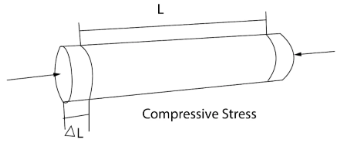#### 2. Tangential or Shearing Stress:

When the deforming force acts tangentially to the surface of a body, it generates a change in the shape of the body. This tangential force applied per unit area is termed as tangential stress or shearing stress.

#### 3. Hydraulic Stress:

When the applied force is due to a liquid uniformly from all sides, then the corresponding stress is termed as hydrostatic stress.

### 8. Strain:

When a deforming force gets applied on an object, the object undergoes a change in its shape and size. The fractional change in their setup is termed a strain.

#### 8.1  Mathematical Equation:

$\text{Strain}=\frac{\text{change in dimension}}{\text{original dimension}}$

It is a dimensionless quantity and has no unit.

According to the change in setup, the strain is differentiated into three types:

$\text{a) Longitudinal strain}=\frac{\text{change in length}}{\text{original length}}$

$\text{b) Volumetric strain}=\frac{\text{change in volume}}{\text{Original volume}}$

$c)\text{ }Shearing\text{ }strain=\frac{tangential\text{ }applied\text{ }force}{Area\text{ }of\text{ }force}$

### 9. Hooke’s Law

Robert Hook observed that within the elastic limit, the stress turns out to be directly proportional to the strain. i.e.,$stress\propto strain\Rightarrow stress=K.strain$

where $K$ is the constant of proportionality known as the ‘Elastic Modulus’ of the material.

Here, it is to be noted that there are some materials that do not obey Hooke’s law like rubber, human’s muscle, etc.

#### 9.1.1  Young’s Modulus of Rigidity $\left( Y \right)$:

It refers to the ratio of normal(longitudinal) stress to the longitudinal strain within the elastic limit.

$Y=\frac{\text{longitudinal stress}}{\text{Longitudinal strain}}$

It has the same unit as stress because strain does not have any unit. Clearly, $Y$ is measured in $N/{{m}^{2}}$ or Pa.

Metals usually have high values of Young’s modulus compared to other materials. Scientifically, the higher Young’s modulus of the material, the more elastic it is.

#### 9.1.2  Bulk Modulus of Rigidity:

It refers to the ratio of direct stress to the volumetric strain within the elastic limit.

$\kappa =\frac{\text{direct stress}}{\text{Volumetric strain}}$

or

$\kappa =\frac{\frac{-F}{A}}{\frac{\Delta V}{V}}=\frac{-PV}{\Delta V}$

The SI unit of bulk modulus is $N/{{m}^{2}}$.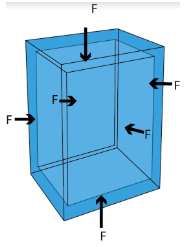#### Compressibility:

The compressibility of a material refers to the reciprocal of its bulk modulus of elasticity. Mathematically, it is given by

$C=\frac{1}{\kappa }$

Its SI unit is ${{N}^{-1}}{{m}^{2}}$ and CGS unit is $dyn{{e}^{-1}}c{{m}^{2}}$.

#### 9.1.3  Modulus of Rigidity or Shear Modulus $(\eta )$:

It refers to the ratio of tangential stress to the shear strain within the elastic limit. Mathematically,

$\eta =\frac{\text{tangential stress}}{\text{shear strain}}$

$\eta =\frac{\frac{F}{A}}{Y}=\frac{F}{AY}$

$\eta =\frac{F}{AY}$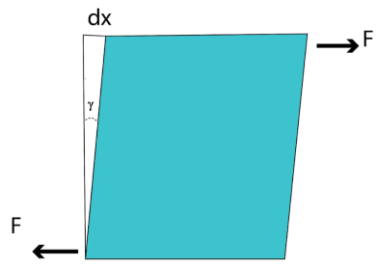The SI unit of shear modulus is $N/{{m}^{2}}$.

Here, it is to be noted that the shear modulus of a material is always considerably smaller than the Young’s modulus

### 10. Limit of Elasticity:

The maximum value of deforming force for which elasticity is experienced in the body is known as its limit of elasticity.

### 11.Stress-Strain Curve: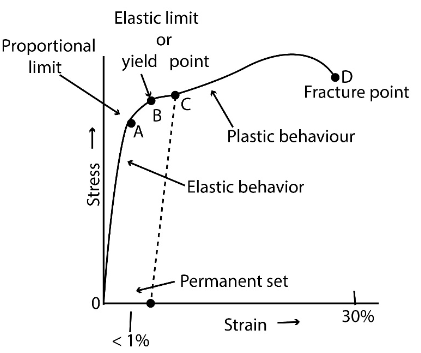Above graph shows the stress-strain curve for a metal wire which is gradually being loaded.

1. The initial part OA of the graph is a straight line expressing that stress is proportional to strain. Up to the point A, Hooke’s law is obeyed. Point A is known as the proportional limit. In this region, the wire is perfectly elastic.

2. After the point A, the stress is not proportional to strain and a curved portion AB is generated. But, if the load is removed at any point between O and B, the curve is retraced along BAO and the wire regains its original length. The portion OB of the graph is known as the elastic region and the point B is termed the elastic limit or yield point. The stress corresponding to B is known as yield strength.

3. Beyond the point B, the strain increases more rapidly than stress. When the load is removed at any point C, the wire cannot come back to its original length but follows the dashed line. Even on decreasing the stress to zero, a residual strain same as OE is left in the wire. Here, the material acquires a permanent set. The fact that the stress-strain curve is not retraced on reversing the strain is termed elastic hysteresis.

4. When the load is increased beyond the point C, there is a large increase in the strain or the length of the wire. In this region, the constrictions (termed necks and waists) develop at some points along the length of the wire and the wire breaks finally at the point D, termed as the fracture point.

In the region between B and D, the length of the wire keeps on increasing even without any addition of load. This region is known as the plastic region and the material undergoes plastic flow or plastic deformation here. The stress corresponding to the breaking point is termed the ultimate strength or tensile strength of the material.

### 12. Elastic after Effect:

Objects return to their original state when deforming force is removed. Certain objects return to their original state immediately after the removal of the deforming force, whereas some objects take longer to do so. The delay in attaining back the original state by an object on the removal of the deforming force is termed an elastic after effect.

### 13. Elastic Fatigue:

The property of an elastic body by virtue of which its behaviour becomes less elastic under the application of repeated alternating deforming force is known as elastic fatigue.

### 14. Ductile Materials:

The materials that have a large plastic range of extension are known as ductile materials. These materials undergo an irreversible rise in their lengths before snapping. Thus, they can be drawn into thin wires. Some examples of ductile materials are copper, silver, iron and aluminium.

### 15. Brittle Materials:

The materials that have a very small range of plastic extension are known as brittle materials. These materials break as soon as the stress is increased beyond the elastic limit. Some examples of brittle materials are cast iron, glass and ceram. ics

### 16. Elastomers:

The materials for which the strain produced is much larger than the stress applied, within the limit of elasticity, are termed elastomers. Some examples of elastomers are rubber, the elastic tissue of the aorta and the large vessel carrying blood from the heart. They have no plastic range.

### 17. Elastic Potential Energy of Stretched Wire:

When a wire is made to stretch, interatomic forces come into play, which opposes the change. Work has to be done against these restoring forces. The work done in stretching the wire is stored in it as its elastic potential energy.

### 18. Poisson’s Ratio:

On the application of a deforming force at the free end of a suspended wire of length $l$ and diameter $D$, its length increases by $\Delta l$ but its diameter decreases by $\Delta D$.

Now, two kinds of strains are produced by a single force:

1. Longitudinal strain $=\frac{\Delta l}{l}$

2. Lateral strain $=\frac{-\Delta D}{D}$

Mathematically,

Poisson’s Ratio $\left( \sigma \right)=\frac{Lateral\text{ }strain}{longitudinal\text{ }strain}=\frac{\left( \frac{-\Delta D}{D} \right)}{\left( \frac{\Delta l}{l} \right)}=-\frac{l\Delta D}{D\Delta l}$

The negative sign shows that longitudinal and lateral strains are opposite in nature.

Because Poisson’s ratio is the ratio of two strains, it has no units and dimensions.

The theoretical value of Poisson’s ratio lies between $-1\text{ and }0.5$  whereas its practical value lies between $0\text{ and }0.5$.

### 19. Applications of Elasticity:

The elastic behavior of materials plays a major role in everyday life. All engineering designs need precise knowledge of the elastic behavior of materials. For instance, while designing a building, the structural design of the columns, beams and supports need ample knowledge of the strength of materials used.

A bridge has to be designed such that it should withstand the load of the flowing traffic, the force of winds as well as its own weight. Likewise, in the design of buildings, usage of beams and columns is popular. In both these cases, the overcoming of the problem of bending of beam under a load is of utmost priority. The beam must not bend too much or break. Now, let us consider the case of a beam loaded at the centre and supported near its ends as shown in the following diagram.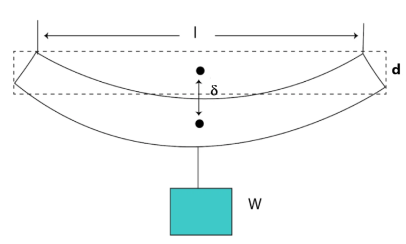A bar of length $l$, breadth $b$, and depth $d$, when loaded at the centre by a load $W$ sinks by an amount given by $\delta =\frac{W{{I}^{3}}}{\left( 4b{{d}^{3}}Y \right)}$

Bending can be limited by using a material with a large Young’s modulus $Y$. Depression can be reduced more effectively by increasing the depth d rather than the breadth $b$. However, a deep bar has a tendency to bend under the weight of moving traffic, thus a better choice is to have a bar of I-shaped cross-section. Such an arrangement gives a large load-bearing surface and ample depth to prevent bending. Also, such a shape reduces the weight of the beam without any sacrifice in its strength and thus reduces the cost.

## FAQs on Mechanical Properties of Solids Class 11 Notes CBSE Physics Chapter 9 (Free PDF Download)

Question 1. Briefly Explain the Different Types of Strain.

Ans: Strain is defined as the change in the shape or size of the body after a deforming force is applied to the body.

1. Longitudinal Strain - Any change in the length of the body is defined as a longitudinal or tensile strain.

Strain = Change in length/Original length = △l/l

1. Volumetric Strain - If the deforming force brings about a change in the volume of the body it acts upon it is defined as the volumetric strain.

Strain = Change in volume/Original volume = △V/V

1. Shear Strain - The tilt in angle caused due to the tangential stress on the body.

Strain = θ = △L/L

Question 2. Explain Poisson’s Ratio.

Ans: Poisson’s ratio talks about the change in the width of the material to the change in the length of the material per unit width and length respectively. Mathematically it can be defined as the negative ratio of a transverse strain to longitudinal strain.

The change in the diameter of the body due to external force applied is the transverse strain and the change in the length of the body is a longitudinal strain.

Poisson’s ratio = σ = -Transverse Strain/Longitudinal strain

σ = -(△D/D)/(△l/l)